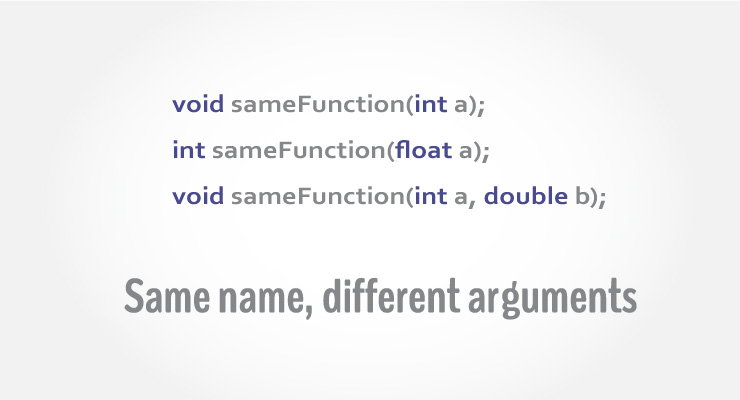Two or more functions having same name but different argument(s) are known as overloaded functions. In this article, you will learn about function overloading with examples.Function refers to a segment that groups code to perform a specific task.

In C++ programming, two functions can have same name if number and/or type of arguments passed are different.

These functions having different number or type (or both) of parameters are known as overloaded functions. For example:

int test() { }
int test(int a) { }
float test(double a) { }
int test(int a, double b) { }

Here, all 4 functions are overloaded functions because argument(s) passed to these functions are different.

Notice that, the return type of all these 4 functions are not same. Overloaded functions may or may not have different return type but it should have different argument(s).

// Error code
int test(int a) { }
double test(int b){ }

The number and type of arguments passed to these two functions are same even though the return type is different. Hence, the compiler will throw error.

#include <iostream>
using namespace std;

void display(int);
void display(float);
void display(int, float);

int main() {

int a = 5;
float b = 5.5;

display(a);
display(b);
display(a, b);

return 0;
}

void display(int var) {
cout << "Integer number: " << var << endl;
}

void display(float var) {
cout << "Float number: " << var << endl;
}

void display(int var1, float var2) {
cout << "Integer number: " << var1;
cout << " and float number:" << var2;
}

Output

Integer number: 5
Float number: 5.5
Integer number: 5 and float number: 5.5

Here, the display() function is called three times with different type or number of arguments.

The return type of all these functions are same but it's not necessary.

// Program to compute absolute value
// Works both for integer and float

#include <iostream>
using namespace std;

int absolute(int);
float absolute(float);

int main() {
int a = -5;
float b = 5.5;

cout << "Absolute value of " << a << " = " << absolute(a) << endl;
cout << "Absolute value of " << b << " = " << absolute(b);
return 0;
}

int absolute(int var) {
if (var < 0)
var = -var;
return var;
}

float absolute(float var){
if (var < 0.0)
var = -var;
return var;
}

Output

Absolute value of -5 = 5
Absolute value of 5.5 = 5.5

In the above example, two functions absolute() are overloaded.

Both functions take single argument. However, one function takes integer as an argument and other takes float as an argument.

When absolute() function is called with integer as an argument, this function is called:

int absolute(int var) {
if (var < 0)
var = -var;
return var;
}

When absolute() function is called with float as an argument, this function is called:

float absolute(float var){
if (var < 0.0)
var = -var;
return var;
}